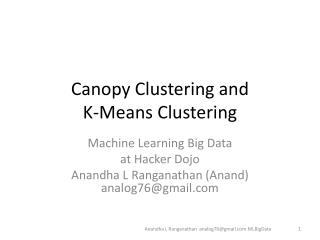DownloadDownload PresentationCanopy Clustering and K-Means Clustering

# Canopy Clustering and K-Means Clustering

Download Presentation## Canopy Clustering and K-Means Clustering

- - - - - - - - - - - - - - - - - - - - - - - - - - - E N D - - - - - - - - - - - - - - - - - - - - - - - - - - -
##### Presentation Transcript

1. Canopy Clustering and K-Means Clustering Machine Learning Big Data at Hacker Dojo Anandha L Ranganathan (Anand)analog76@gmail.com Anandha L Ranganathan analog76@gmail.com MLBigData

2. Movie Dataset • Download the movie dataset from http://www.grouplens.org/node/73 • The data is in the format UserID::MovieID::Rating::Timestamp • 1::1193::5::978300760 • 2::1194::4::978300762 • 7::1123::1::978300760 Anandha L Ranganathan analog76@gmail.com MLBigData

3. Similarity Measure • Jaccard similarity coefficient • Cosine similarity Anandha L Ranganathan analog76@gmail.com MLBigData

4. JaccardIndex • Distance = # of movies watched by by User A and B / Total # of movies watched by either user. • In other words A  B / A  B. • For our applicaton I am going to compare the the subset of user z₁ and z₂ where z₁,z₂ ε Z • http://en.wikipedia.org/wiki/Jaccard_index Anandha L Ranganathan analog76@gmail.com MLBigData

5. Jaccard Similarity Coefficient. similarity(String[] s1, String[] s2){ List<String> lstSx=Arrays.asList(s1); List<String> lstSy=Arrays.asList(s2); Set<String> unionSxSy = new HashSet<String>(lstSx); unionSxSy.addAll(lstSy); Set<String> intersectionSxSy =new HashSet<String>(lstSx); intersectionSxSy.retainAll(lstSy); sim= intersectionSxSy.size() / (double)unionSxSy.size(); } Anandha L Ranganathan analog76@gmail.com MLBigData

6. Cosine Similiarty • distance = Dot Inner Product (A, B) / sqrt(||A||*||B||) • Simple distance calculation will be used for Canopy clustering. • Expensive distance calculation will be used for K-means clustering. Anandha L Ranganathan analog76@gmail.com MLBigData

7. Canopy Clustering- Mapper • Canopy cluster are subset of total popultation. • Points in that cluster are movies. • If z₁subset of the whole population, rated movie M1 and same subset are rated M2 also then the movie M1and M2 are belong the same canopy cluster. Anandha L Ranganathan analog76@gmail.com MLBigData

8. Canopy Cluster – Mapper • First received point/data is center of Canopy . • Receive the second point and if it is distance from canopy center is less than T1 then they are point of that canopy. • If d(P1,P2) >T1 then that point is new canopy center. • If d(P1,P2) < T1 they are point of centroidP1. • Continue the step 2,3,4 until the mappercomplets its job. • Distance is measured between 0 to 1. • T1 value is 0.005 and I expect around 200 canopy clusters. • T2 value is 0.0010. Anandha L Ranganathan analog76@gmail.com MLBigData

9. Canopy Cluster – Mapper • Pseudo Code. booleanpointStronglyBoundToCanopyCenter = false for (Canopy canopy : canopies) { double centerPoint= canopyCenter.getPoint(); if(distanceMeasure.similarity(centerPoint, movie_id) > T1) pointStronglyBoundToCanopyCenter = true } if(!pointStronglyBoundToCanopyCenter){ canopies.add(new Canopy(0.0d)); Anandha L Ranganathan analog76@gmail.com MLBigData

10. Data Massaging • Convert the data into the required format. • In this case the converted data to be displayed in <MovieId,List of Users> • <MovieId, List<userId,ranking>> Anandha L Ranganathan analog76@gmail.com MLBigData

11. Canopy Cluster – Mapper A Anandha L Ranganathan analog76@gmail.com MLBigData

12. Threshold value Anandha L Ranganathan analog76@gmail.com MLBigData

13. Anandha L Ranganathan analog76@gmail.com MLBigData

14. Anandha L Ranganathan analog76@gmail.com MLBigData

15. Anandha L Ranganathan analog76@gmail.com MLBigData

16. Anandha L Ranganathan analog76@gmail.com MLBigData

17. Anandha L Ranganathan analog76@gmail.com MLBigData

18. Anandha L Ranganathan analog76@gmail.com MLBigData

19. ReducerMapper A - Red center Mapper B – Green center Anandha L Ranganathan analog76@gmail.com MLBigData

20. Redundant centers within the threshold of each other. Anandha L Ranganathan analog76@gmail.com MLBigData

21. Add small error => Threshold+ξ Anandha L Ranganathan analog76@gmail.com MLBigData

22. So far we found , only the canopy center. • Run another MR job to find out points that are belong to canopy center. • canopy clusters areready when the job is completed. • How it would look like ? Anandha L Ranganathan analog76@gmail.com MLBigData

23. Canopy Cluster - Before MR jobSparse Matrix Anandha L Ranganathan analog76@gmail.com MLBigData

24. Canopy Cluster – After MR job Anandha L Ranganathan analog76@gmail.com MLBigData

25. Cells with values 1 are grouped together and users are moved from their original location Anandha L Ranganathan analog76@gmail.com MLBigData

26. K – Means Clustering • Output of Canopy cluster will become input of K-means clustering. • Apply Cosine similarity metric to find out similar users. • To find Cosine similarity create a vector in the format <UserId,List<Movies>> • <UserId,{m1,m2,m3,m4,m5}> Anandha L Ranganathan analog76@gmail.com MLBigData

27. Anandha L Ranganathan analog76@gmail.com MLBigData

28. Vector(A) - 1111000 • Vector (B)- 0100111 • Vector (C)- 1110010 • distance(A,B) = Vector (A) * Vector (B) / (||A||*||B||) • Vector(A)*Vector(B) = 1 • ||A||*||B||=2*2=4 •  ¼=.25 • Similarity (A,B) = .25 Anandha L Ranganathan analog76@gmail.com MLBigData

29. Find k-neighbors from the same canopy cluster. • Do not get any point from another canopy cluster if you want small number of neighbors • # of K-means cluster > # of Canopy cluster. • After couple of map-reduce jobs K-means cluster is ready Anandha L Ranganathan analog76@gmail.com MLBigData

30. Find Nearest Cluster of a point - Map Public void addPointToCluster(Point p ,Iterable<KMeansCluster> lstKMeansCluster) { kMeansClusterclosesCluster = null; Double closestDistance = CanopyThresholdT1/3 For(KMeansClustercluster :lstKMeansCluster){ double distance=distance(cluster.getCenter(),point) if(closesCluster || closestDistance >distance){ closesetCluster= cluster; closesDistance= distance } } closesCluster.add(point); } Anandha L Ranganathan analog76@gmail.com MLBigData

31. Find convergence and Compute Centroid - Reduce Public void computeConvergence((Iterable<KMeansCluster> clusters){ for(Cluster cluster:clusters){ newCentroid = cluster.computeCentroid(cluster); if(cluster.getCentroid()==newCentroid){ cluster.converged=true; } else { cluster.setCentroid(newCentroid) } } • Run the process to find nearest cluster of a point and centroid until the centroidbecomes static. Anandha L Ranganathan analog76@gmail.com MLBigData

32. All points –before clustering Anandha L Ranganathan analog76@gmail.com MLBigData

33. Canopy - clustering Anandha L Ranganathan analog76@gmail.com MLBigData

34. Canopy Clusering and K means clustering. Anandha L Ranganathan analog76@gmail.com MLBigData

35. ? Anandha L Ranganathan analog76@gmail.com MLBigData